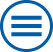## The group contributes to the following mathematical research topics of WIAS:

Methods for optimal stopping and control

Stochastic numerical algorithms for optimal stopping and control problems are required for the evaluation of usually high-dimensional callable or cancelable products, or the determination of optimal decision strategies in systems involving high-dimensional underlying quantities. In this respect, primal methods provide suboptimal exercise strategies, hence lower estimations of the target value (e.g. price), while dual methods provide upper estimations. Naturally, the gap between lower and upper bounds due to these approaches should be as small as possible. [>> more]

Statistical Inference

The term statistical inference summerizes methods to extract information from observed data in order to characterize properties in populations. This involves statistical modeling, estimation and uncertainty assessment of parameters, testing of hypothesis. [>> more]

Stochastic Optimization

Stochastic Optimization in the widest sense is concerned with optimization problems influenced by random parameters in the objective or constraints. [>> more]

Variational methods

Many physical phenomena can be described by suitable functionals, whose critical points play the role of equilibrium solutions. Of particular interest are local and global minimizers: a soap bubble minimizes the surface area subject to a given volume and an elastic body minimizes the stored elastic energy subject to given boundary conditions. [>> more]